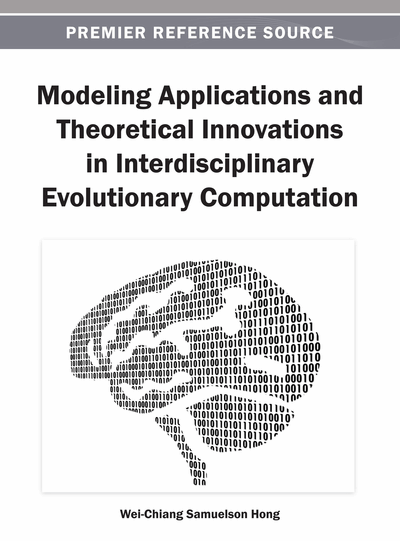# An Evolutionary Functional Link Neural Fuzzy Model for Financial Time Series Forecasting

S. Chakravarty, P. K. Dash, V. Ravikumar Pandi, B. K. Panigrahi
DOI: 10.4018/978-1-4666-3628-6.ch012
OnDemand:
(Individual Chapters)
Available
\$37.50
No Current Special Offers

## Abstract

This paper proposes a hybrid model, evolutionary functional link neural fuzzy model (EFLNF), to forecast financial time series where the parameters are optimized by two most efficient evolutionary algorithms: (a) genetic algorithm (GA) and (b) particle swarm optimization (PSO). When the periodicity is just one day, PSO produces a better result than that of GA. But the gap in the performance between them increases as periodicity increases. The convergence speed is also better in case of PSO for one week and one month a head prediction. To testify the superiority of the EFLNF, a number of comparative studies have been made. First, functional link artificial neural network (FLANN) and functional link neural fuzzy (FLNF) were combined with back propagation (BP) learning algorithm. The result shows that FLNF performs better than FLANN. Again, FLNF is compared with EFLNF where the latter outperforms the former irrespective of the periodicity or the learning algorithms with which it has been combined. All models are used to predict the most chaotic financial time series data; BSE Sensex and S&P CNX Nifty stock indices one day, one week and one month in advance.
Chapter Preview
Top

## 1. Introduction

The chaotic, volatile and random nature of stock market has remained as main hurdle in forecasting. As an institution, it is not only impacted by macro-economic factors but also political development of a country, strategic planning of corporate houses and above all, mood of individual investors. However, over the years efficient and intelligent models have been developed by researchers to forecast it.

Broadly, there are two types of models for time series forecasting: (1) linear models, e.g. moving average, exponential smoothing, autoregressive moving average (ARMA) and autoregressive integrated moving average (ARIMA); (2) non-linear models, e.g. neural network models, support vector machine, fuzzy system models etc. For better result, researchers have also developed hybrid models integrating both linear and nonlinear models (Valenzuelaa et al., 2007; Khashei, Bijari, & Ardali, 2009). Secondly, hybrid models have also been developed combining non-linear models only and different evolutionary learning algorithms like genetic algorithm (GA) and particle swarm optimization (PSO) technique. A survey of literature will show this clearly.

A local linear wavelet neural network (LLWNN) (Chen, Yang, & Dong, 2006) is used to predict Box-Jenkins and Mackey glass time series where a hybrid training algorithm of particle swarm optimization and gradient descent method is introduced to train the model. Application of the same model is seen (Chen, Dong, & Zhao, 2005) to predict stock market indices where the parameters are optimized by using estimation of distribution algorithm. A combination of wavelet and Takagi Sugeno Kang (TSK) fuzzy rules based system is applied (Chang, Fan, & Chen, 2007) to predict financial time series data of Taiwan stock market. A fuzzy time series method based on a multiple-period modified equation derived from adaptive expectation model (Cheng, Chen, & Teoh, 2007) is used to forecast, taking the same data set. Support vector machine (Huang, Nakamori, & Wang, 2004) is used to forecast stock movement direction for NIKKEI 225 index. A least square support vector machine with a mixed kernel where genetic algorithm is used to select input features and another GA used for parameters optimization (Yu, Chen, Wang, & Lai, 2009) to predict S&P 500, DJIA and New York stock exchange. Kim and Han (2000) have applied a hybrid model, combination of artificial neural network (ANN) and GA to predict stock price indices where GA works to reduce the dimension of ANN and determine the connection weights (Kim & Han, 2000). A linear combinatory with adaptive bacterial foraging optimization is used (Majhi, Panda, Majhi, & Sahoo, 2009) to predict stock market indices. A single multiplicative neuron with cooperative random learning particle swarm optimization is applied (Zhao & Yang, 2009) to predict Mackey glass time series. A hybrid forecast method, combination of fuzzy time series and particle swarm optimization technique is used (Kuo et al., 2009) to forecast Taiwan stock exchange.

## Complete Chapter List

Search this Book:
Reset